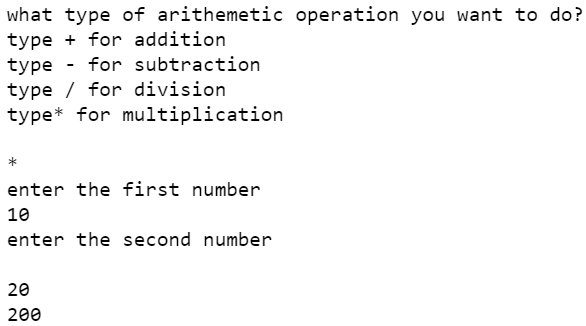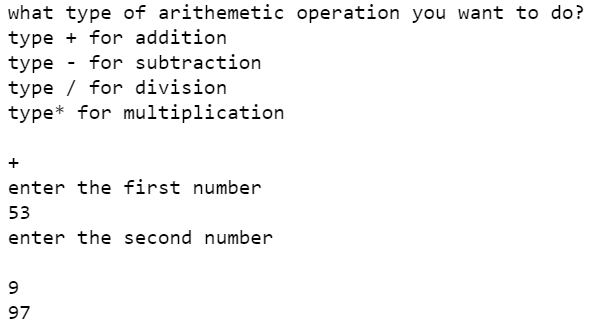Related Articles

# Faulty calculator using Python

• Difficulty Level : Easy
• Last Updated : 01 Jul, 2021

A faulty calculator is simply a calculator which operates simple tasks, but in some cases (set by the programmer) it gives the wrong output. You all must be wondering why do we need a faulty calculator? This type of calculator is not needed unless you want to prank someone or prove them wrong in case of some maths problem.

Approach:

• First, we take input from the user that what he/she wants to do
• Then we write the code of Addition, Subtraction, Multiplication, and Division
• And lastly, we insert those cases that we want wrong results.

Implementation:

## Python

 `print``(``"what type of arithmetic operation you want to do?\n"``      ``"type + for addition\n"``      ``"type - for subtraction\n"``      ``"type / for division\n"``      ``"type* for multiplication\n"``)`  `# taking input``type_of_calculation ``=` `input``()` `print``(``"enter the first number"``)``A ``=` `int``(``input``())` `print``(``"enter the second number\n"``)``B ``=` `int``(``input``())` `b ``=` `"+"``c ``=` `"-"``d ``=` `"*"``e ``=` `"/"`  `# setting normal and false condition for calculator``if` `type_of_calculation ``=``=` `b:``    ``# for addition``    ``if` `(A ``=``=` `53` `and` `B ``=``=` `9``) ``or` `(A ``=``=` `90` `and` `B ``=``=` `52``):``        ``print``(``97``)``    ``else``:``        ``print``(A``+``B)``elif` `type_of_calculation ``=``=` `c:``  ` `    ``# for subtraction``    ``print``(A``-``B)``elif` `type_of_calculation ``=``=` `d:``  ` `    ``# for multiplication``    ``if` `A ``=``=` `45` `and` `B ``=``=` `3` `or` `A ``=``=` `4` `and` `B ``=``=` `67``:``        ``print``(``575``)``    ``else``:``        ``print``(A``*``B)``elif` `type_of_calculation ``=``=` `e:``  ` `    ``# for division``    ``if` `A ``=``=` `5` `and` `B ``=``=` `63``:``        ``print``(``40``)``    ``else``:``        ``print``(A``/``B)`

Output:

For normal conditionFor false conditionAttention reader! Don’t stop learning now. Participate in the Scholorship Test for First-Step-to-DSA Course for Class 9 to 12 students.

My Personal Notes arrow_drop_up